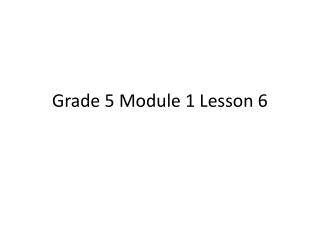DownloadDownload PresentationGrade 5 Module 1 Lesson 6

# Grade 5 Module 1 Lesson 6

Download Presentation## Grade 5 Module 1 Lesson 6

- - - - - - - - - - - - - - - - - - - - - - - - - - - E N D - - - - - - - - - - - - - - - - - - - - - - - - - - -
##### Presentation Transcript

1. Grade 5 Module 1 Lesson 6

2. Find the Midpoint

3. Find the Midpoint 0 1 0.5

4. Find the Midpoint 0 0.1

5. Find the Midpoint 0 0.1 0.05

6. Find the Midpoint 0 0.01

7. Find the Midpoint 0 0.01 0.005

8. Raname the Units 100 cm = ___ m 200 cm = ___ m 700 cm = ___ m 750 cm = ___ m ___ cm

9. Raname the Units 100 cm = 1 meters 200 cm = 2 meters 700 cm = 7 meters 750 cm = 7 meters 50 cm

10. Multiply by Decimal Fractions Say the multiplication sentence. 3 x 10 =

11. Multiply by Decimal Fractions Write the 3 in the Tens column. 3 x 10 =___

12. Multiply by Decimal Fractions Say the multiplication sentence. 4x1= __

13. Multiply by Decimal Fractions Write 4 in the ones column and fill in the addition sentence so that it reads 30 + 4.

14. Multiply by Decimal Fractions Say the multiplication Sentence. 6 x 1/10 =

15. Multiply by Decimal Fractions Write the 6 in the tenths place. 6 x 1/10 =__

16. Multiply by Decimal Fractions Say the multiplication Sentence. 5 x 1/100 =

17. Multiply by Decimal Fractions Say the multiplication sentence. 2 x 1/1000 =

18. Multiply by Decimal Fractions Write the 2 in the thousandths place. 2 x 1/1000 =__

19. Multiply by Decimal Fraction Say the addition sentence.

20. Multiply by Decimal Fraction Say the addition sentence. 30 + 4 + 0.6 + 0.05 + 0.002 = 34.652

21. Concept Development Problem 1 Use your Place Value Chart to Compare 13,196 and 13,296 Read the first number. Read the second number Which number is larger? How can you tell? Use a symbol to show which number is greater.

22. Concept Development 13,296 is larger than 13,196 because the digit in the hundreds place is one bigger. 13,296 is 100 more than 13,196. 13,196 < 13,296

23. Concept Development Write 2 thousandths in standard form on your place value chart. Say the digit that you wrote on your chart.

24. Concept Development Write 2 thousandths in standard form on your place value chart. Say the digit that you wrote on your chart. -- Zero point zero zero two

25. Concept Development Problem 2 Compare 0.012 and 0.002

26. Concept Development Write 12 thousandths in standard form underneath 0.002 on your chart. Say the digits that you wrote on your chart.

27. Concept Development Write 12 thousandths in standard form underneath 0.002 on your chart. Say the digits that you wrote on your chart. Zero point zero one two Say this number in unit form.

28. Concept Development Write 12 thousandths in standard form underneath 0.002 on your chart. Say the digits that you wrote on your chart. Zero point zero one two Say this number in unit form. 1 hundredth 2 thousandths

29. Concept Development Which number is larger? Turn and talk to your partner about how you can decide.

30. Concept Development Which number is larger? Turn and talk to your partner about how you can decide. 0.002 < 0.012

31. Concept Development Problem 3 Compare 299/1000 and 3/10

32. Concept Development Problem 3 Write 3 tenths in standard form on your place value chart.

33. Concept Development Problem 3 Write 299 thousandths in standard form on your place value chart under 3 tenths.

34. Concept Development Problem 3 Which decimal has more tenths?

35. Concept Development Problem 3 Which decimal has more tenths? 0.3

36. Concept Development Problem 3 If we traded 3 tenths for thousandths, how many thousandths would we need? Turn and talk to your partner.

37. Concept Development Problem 3 If we traded 3 tenths for thousandths, how many thousandths would we need? 300 thousandths

38. Concept Development Problem 3 Name these decimals using unit form and compare. Tell your partner which is more.

39. Concept Development Problem 3 Unit Form 299 thousandths; 300 thousandths 300 thousandths is more 0.299 < 0.3

40. Concept Development Problem 4 Order from least to greatest: 0.413, 0.056, 0.164, and 0.531 Explain the strategy you used

41. Problem Set • Do you personal best to complete the problem set within the allotted 10 minutes. Do problems 1,2, and 5 first. Leave 3 and 6 to the end if you still have time.

42. Student Debrief Lesson Objective: Compare decimal fractions to the thousandths using like units and express comparisons with >, <, =.